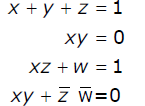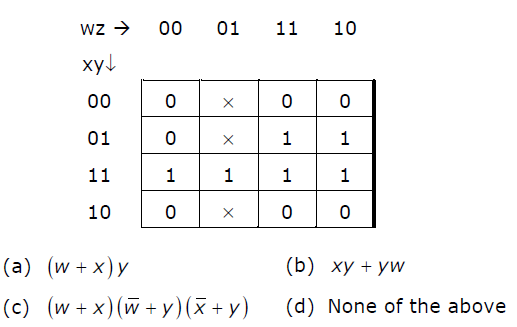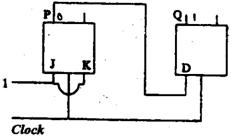51. The complement of the function F = (A + B’)(C’ + D)(B’ + C) is: a. A’B + CD’ + BC' b. AB’ + C’D + B’C c. AB’ + CD’ + BC d. AB + BC + CD
 Answer: (a).A’B + CD’ + BC'

 52. To put the 8085 microprocessor in the wait state a. lower the-HOLD input b. lower the READY input c. raise the HOLD input d. raise the READY input

 53. Comparing the time T1 taken for a single instruction on a pipelined CPU with time T2 taken on a non­ pipelined but identical CPU, we can say that a. T1 <= T2 b. T1 >= T2 c. T1 < T2 d. T1 is T2 plus the time taken for one instruction fetch cycle

 54. The 8085 microprocessor responds to the presence of an interrupt a. As soon as the TRAP pin becomes 'high' b. By checking the TRAP pin for 'high' status at the end of each instruction fetch c. By checking the TRAP pin for 'high' status at the end of the execution of each instruction d. By Checking the TRAP pin for 'high' status at regular intervals
 Answer: (c).By checking the TRAP pin for 'high' status at the end of the execution of each instruction

 55. The most appropriate matching for the following pairsX: Indirect addressing 1 : LoopsY: Immediate addressing 2 : PointersZ: Auto decrement addressing 3: Constantsis a. X-3, Y-2, Z-1 b. X-I, Y-3, Z-2 c. X-2, Y-3, Z-1 d. X-3, Y-l, Z-2

 56. The simultaneous equations on the Boolean variables x, y, z and w, have the following solution for x, y, z and w, respectively.a. 0 1 0 0 b. 1 1 0 1 c. 1 0 1 1 d. 1 0 0 0

 57. Which function does NOT implement the Karnaugh map given below?a. a b. b c. c d. d
 58. The following arrangement of master-slave flip flops has the initial state of P, Q as 0, 1 (respectively). After three clock cycles the output state P, Q is (respectively),a. 1, 0 b. 1, 1 c. 0, 0 d. 0,1